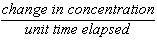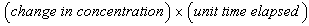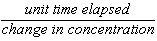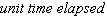# Kinetics Practice Test Multiple Choice

 Page 1/3 Date 23.04.2018 Size 272.25 Kb.
1   2   3
Kinetics Practice Test
Multiple Choice

Identify the choice that best completes the statement or answers the question.
____ 1. The equation H2(g) + I2(g) 2HI(g) is a(n)
 a. overall reaction. c. reaction pathway. b. reaction mechanism. d. intermediate reaction.

____ 2. What is the sequence of steps in a reaction called?

 a. heterogeneous reaction c. overall reaction b. rate law d. reaction mechanism

____ 3. Most steps in a reaction mechanism

 a. are complicated. c. are easily observable. b. involve several molecules. d. are simple.

____ 4. In the reaction represented by the following equation, a possible intermediate is H2(g) + I2(g) 2HI(g)

 a. H2. c. H2I. b. HI. d. I2.

____ 5. Reactions whose reactants and products exist in a single phase are called

 a. reaction mechanisms. c. homogeneous reactions. b. heterogeneous reactions. d. activated complexes.

____ 6. To react, gas particles must

 a. be in the same physical state. c. have different energies. b. have the same energy. d. collide.

____ 7. To be effective, a collision requires

 a. sufficient energy. b. a favorable orientation. c. sufficient energy and a favorable orientation. d. a reaction mechanism.

____ 8. Which attempts to explain chemical reactions and physical interactions of molecules?

 a. chemical kinetics c. thermodynamics b. collision theory d. thermochemistry

____ 9. Raising the temperature of gas particles

 a. increases both collision energy and favorability of orientation. b. increases neither collision energy nor favorability of orientation. c. increases collision energy but does not increase favorability of orientation. d. increases favorability of orientation but does not increase collision energy.

____ 10. If a collision between molecules is very gentle, the molecules are

 a. more likely to be favorably oriented. b. less likely to be favorably oriented. c. more likely to react. d. more likely to rebound without reacting.

____ 11. The minimum energy required for an effective collision is called

 a. energy of enthalpy. c. free energy. b. activation energy. d. kinetic energy.

____ 12. A short-lived structure formed during a collision is a(n)

 a. reagent. c. activated complex. b. catalyst. d. inhibitor.

____ 13. The bonding of the activated complex is characteristic of

 a. reactants only. c. both reactants and products. b. products only. d. solids only.

____ 14. An activated complex

 a. always separates into the products. b. always re-forms the reactants. c. may either separate into the products or re-form the reactants. d. always evaporates.

____ 15. In a diagram of an activated complex, broken lines represent

 a. actual bonds. c. electrons. b. partial bonds. d. bond energies.

____ 16. What takes place in an activated complex?

 a. Bonds form. b. Bonds break. c. Some bonds form and other bonds break. d. A catalyst is produced.

____ 17. Raising the temperature of reactants in a system

 a. increases the average kinetic energy of the molecules. b. decreases the average kinetic energy of the molecules. c. decreases the rate of collision of molecules. d. has no effect on the average kinetic energy of molecules.

____ 18. If a collision between molecules is oriented properly, the molecules are

 a. more likely to react. c. less likely to form an intermediate. b. more likely to rebound without interacting. d. less likely to react.

____ 19. Which statement correctly describes the energy changes that occur when bonds form and when bonds break?

 a. Breaking bonds is endothermic, and forming bonds is exothermic. b. Breaking bonds is exothermic, and forming bonds is endothermic. c. Both are exothermic. d. Both are endothermic.

____ 20. is positive for

 a. an endothermic reaction. c. an inhibited reaction. b. an exothermic reaction. d. a stable reaction.

____ 21. Which term has the same numerical value for the forward reaction as it has for the reverse reaction but with opposite sign?

 a. E c. Ea´ b. d. Ea

____ 22. Which of the following is true in an endothermic reaction?

 a. energy of products < activation energy < energy of reactants b. energy of reactants < activation energy < energy of products c. energy of products < energy of reactants < activation energy d. energy of reactants < energy of products < activation energy

____ 23. Which branch of chemistry studies reaction rates?

 a. thermochemistry c. chemical kinetics b. thermodynamics d. calorimetry

____ 24. Which branch of chemistry studies reaction mechanisms?

 a. thermochemistry c. chemical kinetics b. thermodynamics d. calorimetry

____ 25. The usual condition for reaction, a favorable orientation, is not necessary for

 a. synthesis reactions. c. single-displacement reactions. b. decomposition reactions. d. double-displacement reactions.

____ 26. How fast something changes with time is a(n)

 a. area. c. order. b. rate. d. collision.

____ 27. Reaction rate depends upon

 a. both collision frequency and efficiency. b. average kinetic energy. c. collision efficiency. d. average potential energy.

____ 28. Which of the following affects reaction rate?

 a. the nature of reactants c. temperature b. surface area of reactants d. All of the above

____ 29. The decrease in reactant concentration per unit time in a reaction is a measure of the

 a. reaction rate. c. overall reaction. b. heterogeneous reaction. d. reaction mechanism.

____ 30. If the surface area of reactants is larger,

 a. the reaction rate is generally higher. b. the reaction rate is generally lower. c. the reaction rate is not affected. d. the rate-determining step is eliminated.

____ 31. In heterogeneous reactions, the reactants

 a. have unequal masses. c. have unequal volumes. b. are not equally reactive. d. are in different phases.

____ 32. If the temperature of the reactants is lower,

 a. the reaction rate is generally higher. b. the reaction rate is generally lower. c. the reaction rate is not affected. d. the rate-determining step is eliminated.

____ 33. Changing the pressure of a gas is another way of changing its

 a. temperature. c. surface area. b. concentration. d. composition.

____ 34. Doubling the pressure of a gas

 a. increases the concentration by a factor of two. b. reduces the concentration by a factor of two. c. doesn't affect the concentration. d. reduces the concentration by a factor of four.

____ 35. Which process is used to speed up chemical reactions?

 a. calorimetry c. activation b. catalysis d. inhibition

____ 36. Catalysts generally affect chemical reactions by

 a. increasing the temperature of the system. b. increasing the surface area of the reactants. c. providing an alternate pathway with a lower activation energy. d. providing an alternate pathway with a higher activation energy.

____ 37. A substance that slows down chemical processes is called a(n)

 a. inhibitor. c. catalyst. b. reactant. d. indicator.

____ 38. How is a heterogeneous catalyst different from the reactants in a chemical reaction?

 a. The mass of the catalyst is different. b. The chemical properties of the catalyst are different. c. The energy of the catalyst is different. d. The phase of the catalyst is different.

____ 39. In a net equation, catalysts

 a. are shown with the reactants. b. are shown with the products. c. are shown with both reactants and products. d. are not shown.

____ 40. A rate law relates

 a. reaction rate and temperature. b. reaction rate and concentrations of reactants. c. temperature and concentrations of reactants. d. energy and concentrations of reactants.

____ 41. The letter R in a rate law stands for

 a. a proportionality constant. c. temperature. b. concentration. d. reaction rate.

____ 42. The value of k in a rate law

 a. is the same under all conditions. c. varies with time. b. varies with concentration. d. varies with temperature.

____ 43. How are the units for a rate always expressed?

 a.b.c.d.____ 44. In the rate law for the following equation, R=k[A]n[B]m, the units for [A] and [B] are

 a. g-1s-1. c. M. b. M-1s-1. d. Ms-1.

____ 45. In the rate law for the following equation, R=k[A]n[B]m, the unit for R is

 a. g-1s-1. c. M. b. M-1s-1. d. Ms-1.

____ 46. A reaction is zero order in reactant A and second order in reactant B. What happens to the reaction rate when the concentrations of both reactants are doubled?

 a. The reaction rate remains the same. b. The reaction rate increases by a factor of two. c. The reaction rate increases by a factor of four. d. The reaction rate increases by a factor of eight.

____ 47. The rate law for a reaction generally depends most directly on the

 a. net chemical reaction. c. rate-determining step. b. first step in the reaction pathway. d. last step in the reaction pathway.

____ 48. The rate for a reaction between reactants L, M, and N is proportional to the cube of [L] and the square of [M]. What is the rate law for this reaction?

 a. R = k[3L][2M] c. R = k[L]3[M]2 b. R = k[L]3[M]2[N] d. R = k[3L][2M][N]

____ 49. A transition structure that results from an effective energetic collision between reactants is the —

 a. order of the reaction b. rate law c. overall reaction d. activated complex

____ 50. How does the potential energy of the activated complex compare with the energies of the reactants and products?

 a. It is less than both the energy of the reactants and the energy of the products. b. It is less than the energy of the reactants but greater than the energy of the products. c. It is greater than the energy of the reactants but less than the energy of the products. d. It is greater than both the energy of the reactants and the energy of the products.

____ 51. If a collision between molecules is very gentle, the molecules are —

 a. more likely to be oriented favorably b. less likely to be oriented favorably c. likely to react d. likely to rebound without reacting

____ 52. Which of the explanations below describes why catalysts can increase reaction rates?

 a. Adding a catalyst adds more molecules. b. Adding a catalyst adds heat to the reaction. c. Adding a catalyst adds electricity to the reaction. d. Adding a catalyst provides an alternate reaction mechanism having a lower potential-energy barrier between reactants and products.

____ 53. A rate law relates —

 a. reaction rate and temperature b. reaction rate and concentration c. temperature and concentration d. energy and concentration

____ 54. A certain reaction is zero order in reactant A and second order in reactant B. What happens to the reaction rate when the concentrations of both reactants are doubled?

 a. The reaction rate remains the same. b. The reaction rate increases by a factor of two. c. The reaction rate increases by a factor of four. d. The reaction rate increases by a factor of eight.

Directory: site -> handlers